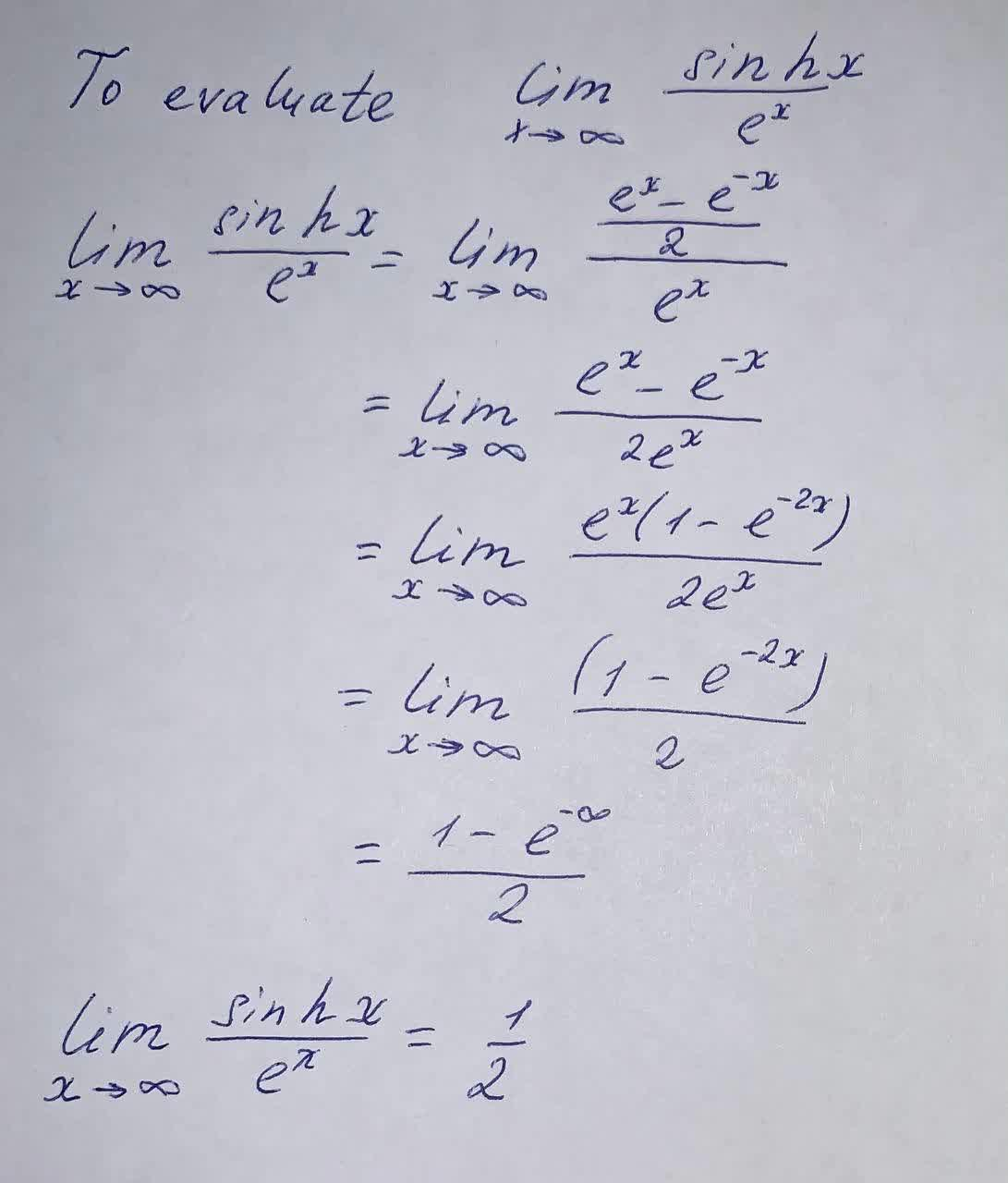Question# Evaluate \lim_{x \rightarrow \infty} \frac{\sin h x}{e^x}

Limits and continuity
ANSWEREDEvaluate $$\lim_{x \rightarrow \infty} \frac{\sin h x}{e^x}$$